Want to share your content on R-bloggers? click here if you have a blog, or here if you don't.

In my last post I worked on two functions that took pairs of variables from a dataset and produced some nice useful `ggplot` plots from them. We started with the simplest case, plotting counts of how two variables cross-tabulate. Then we worked our way up to being able to automate the process of plotting lots of pairings of variables from the same dataframe. We added a feature to change the plot type and tested for general functionality. To be honest I thought I was in great shape until I went and started trying the function on a much larger dataset. Performance was terrible I had made a couple of mistakes at least. Today I’ll fix those problems and combine our two functions into one function.

I’m going to output all the plots in a smaller size for the benefit of you the readers. I’m doing that via RMarkdown and it won’t happen automatically for you if you download and use the code. I’ll be using , fig.width=4.5, fig.height=2

## Background and catch-up

Some quick setup. In a few paragraphs I’ll add lines to the function so you don’t have to run the setup code, but for now…

```library(dplyr)
library(ggplot2)
library(microbenchmark)
theme_set(theme_bw()) # set theme to my personal preference
```

We originally started with a simple task using `dplyr` and `ggplot2` in the console. Take two of the `mtcars` variables, in this case `am` and `cyl`, and conduct a cross tabulation and then plot it. Since it’s the sort of thing I’m likely to do often seemed like a good candidate to write a function for.

```### with dplyr and ggplot manually
mtcars %>%
filter(!is.na(am), !is.na(cyl))  %>%
group_by(am,cyl) %>%
count() %>%
ggplot(aes(fill=am, y=n, x=cyl)) +
geom_bar(position="dodge", stat="identity")
```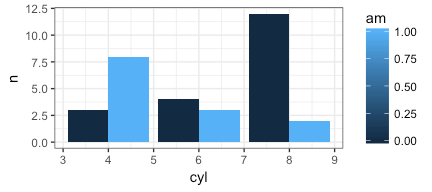By the end of the last post we had accomplished the following tasks:

1. Our function (called `PlotMe`) was a lot more robust. It checked for basic user entry errors, added a useful plot title and generally did what we wanted.
2. Along the way we learned the “tricks” of working with `dplyr` and `ggplot2` inside of functions.
3. It allowed the user to choose the plot type from among three options
4. We could feed it a pair of bare variable names e.g. `am` & `vs` or through a helper we could also feed it multiple column numbers from the data frame and it would produce plots of all the combinations.

Here’s the `PlotMeX` function as we left it (with error checking removed for clarity). If you’re not familiar with `enquo` or the `!!` notation please refer back to an earlier post here.

```PlotMeX <- function(dataframe, x, y, plottype = "side"){
switch(plottype,
side =  list(geom_bar(position="dodge", stat="identity"),
ylab("Count")) -> whichbar,
stack = list(geom_bar(stat="identity"),
ylab("Count")) -> whichbar,
percent = list(geom_bar(stat="identity", position="fill"),
ylab("Percent")) -> whichbar
)

aaa <- enquo(x)
bbb <- enquo(y)
dfname <- enquo(dataframe)
dataframe %>%
filter(!is.na(!! aaa), !is.na(!! bbb))  %>%
mutate(!!quo_name(aaa) := factor(!!aaa), !!quo_name(bbb) := factor(!!bbb)) %>%
group_by(!! aaa,!! bbb) %>%
count() %>%
ggplot(aes_(fill=aaa, y=~n, x=bbb)) +
whichbar +
ggtitle(bquote("Crosstabs"*.(dfname)*.(aaa)~"by"*.(bbb))) -> p
plot(p)
}
PlotMeX(mtcars, am, vs, "stack")
```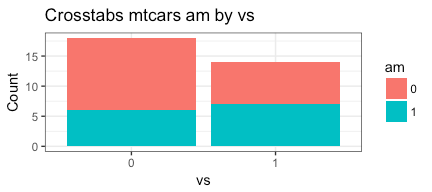```PlotMeX(mtcars, am, vs, "percent")
```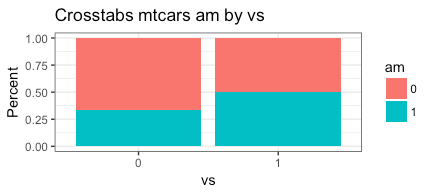```PlotMeX(esoph, alcgp, tobgp, "side")
```As you can see it works fine as far as we can tell on the `mtcars` data set as well as the `esoph` dataset (which is also smallish). The problem I discovered was that it was gross in performing on bigger datasets. Especially if I fed it to `mapply`. Made no sense since I was not plotting a full dataset I was plotting the crosstab data which is already summarized and doesn’t have a lot of rows or columns. Something was wrong.

## What went wrong

Turns out I made two mistakes. Both of them had to do with how much data I was accumulating especially over larger data sets or over repetitions. 3 keys tools may help you figure these things out. `microbenchmark`, `benchplot` and just good old fashioned inspection of what the results the function returns are.

```# deliberately surpressing the figures!
microbenchmark(PlotMeX(mtcars, am, cyl), times = 10)

## Unit: milliseconds
##                      expr      min       lq     mean   median       uq
##  PlotMeX(mtcars, am, cyl) 244.5679 254.0199 255.1998 256.5568 257.5296
##       max neval
##  262.8945    10

benchplot(mtcars %>%
filter(!is.na(am), !is.na(cyl))  %>%
group_by(am,cyl) %>%
count() %>%
ggplot(aes(fill=am, y=n, x=cyl)) +
geom_bar(position="dodge", stat="identity"))

##        step user.self sys.self elapsed
## 1 construct     0.005    0.000   0.005
## 2     build     0.019    0.000   0.020
## 3    render     0.150    0.003   0.157
## 4      draw     0.065    0.009   0.095
## 5     TOTAL     0.239    0.012   0.277

# don't forget to check your "returns"
inspectthisobject <- PlotMeX(mtcars, am, cyl)
```

In my case I decided to play it extra safe and add a intermediate dataframe called `tempdf`. That way it would be much harder to ever accidentally return too much data no matter how I called or invoked the function.

```    count() -> tempdf
tempdf %>%
```

But the real culprit was `dfname <- enquo(dataframe)` which should have been `dfname <- deparse(substitute(dataframe))` or you run the risk of passing back way too much data within the subsequent `ggtitle(bquote("Crosstabs"*.(dfname)*.(aaa)~"by"*.(bbb)))`.

Okay, now our `PlotMeX` has become…

```PlotMeX <- function(dataframe, x, y, plottype = "side"){
switch(plottype,
side =  list(geom_bar(position="dodge", stat="identity"),
ylab("Count")) -> whichbar,
stack = list(geom_bar(stat="identity"),
ylab("Count")) -> whichbar,
percent = list(geom_bar(stat="identity", position="fill"),
ylab("Percent")) -> whichbar
)

aaa <- enquo(x)
bbb <- enquo(y)
xname <- deparse(substitute(x))
yname <- deparse(substitute(y))
dfname <- deparse(substitute(dataframe))
dataframe %>%
filter(!is.na(!! aaa), !is.na(!! bbb))  %>%
mutate(!!quo_name(aaa) := factor(!!aaa), !!quo_name(bbb) := factor(!!bbb)) %>%
group_by(!! aaa,!! bbb) %>%
count() -> tempdf
tempdf %>%
ggplot(aes_(fill=aaa, y=~n, x=bbb)) +
whichbar +
ggtitle(bquote("Crosstabs "*.(dfname)~.(xname)~"by "~.(yname))) -> p
plot(p)
return(dfname)
}
PlotMeX(mtcars,am,vs)
``````##  "mtcars"

PlotMeX(mtcars,am,vs, "stack")
``````##  "mtcars"

PlotMeX(mtcars,am,vs, "percent")
``````##  "mtcars"
```

That’s better. To summarize we now have `PlotMeX` which does our plotting, the `CrossXYs` function which generates lists of things to be plotted, and our error-checking code for user input that I have been ignoring for awhile now. To remind you here’s `CrossXYs`.

```CrossXYs <- function(dataframe, xwhich, ywhich){
# Build two vectors
indvars<-list() # create empty list to add to
depvars<-list() # create empty list to add to
totalcombos <- 1 # keep track of where we are
message("Creating the variable pairings...")
for (j in seq_along(xwhich)) {
for (k in seq_along(ywhich)) {
depvars[[totalcombos]] <- as.name(colnames(dataframe[xwhich[[j]]]))
indvars[[totalcombos]] <- as.name(colnames(dataframe[ywhich[[k]]]))
cat("Pairing #", totalcombos, " ", as.name(colnames(dataframe[xwhich[[j]]])),
" with ", as.name(colnames(dataframe[ywhich[[k]]])), "\n", sep = "")
totalcombos <- totalcombos +1
}
}
return(invisible(list(depvars=depvars,indvars=indvars)))
}
CrossXYs(mtcars,c(10:11),c(2,9))

## Creating the variable pairings...

## Pairing #1 gear with cyl
## Pairing #2 gear with am
## Pairing #3 carb with cyl
## Pairing #4 carb with am
```

## Consolidate for success

My original plan had been to leave them as separate functions and use `mapply` to invoke a multiplot option. But the more I thought about it the more I liked the idea of one function that took care of everything. So what I needed to do was combine the two and figure out from what the user supplies which path to pursue. Here’s the finished product. For now (as we troubleshoot) I have programmed it to return the arguments it was passed. If you want to refresh your memory on the error checking pieces please refer to this post.

```PlotMeX <- function(dataframe, xwhich, ywhich, plottype = "side"){
# error checking
if (!require(ggplot2)) {
}
theme_set(theme_bw())
if (!require(dplyr)) {
}
if (length(match.call()) <= 3) {
stop("Not enough arguments passed... requires a dataframe, plus at least two variables")
}
argList <-  as.list(match.call()[-1])
if (!exists(deparse(substitute(dataframe)))) {
stop("The first object in your list does not exist. It should be a dataframe")
}
if (!is(dataframe, "data.frame")) {
stop("The first name you passed does not appear to be a data frame")
}
switch(plottype,
side =  list(geom_bar(position="dodge", stat="identity"),
ylab("Count")) -> whichbar,
stack = list(geom_bar(stat="identity"),
ylab("Count")) -> whichbar,
percent = list(geom_bar(stat="identity", position="fill"),
ylab("Percent")) -> whichbar
)
# If both variables are found in the dataframe immediately print the plot
if (deparse(substitute(xwhich)) %in% names(dataframe) & deparse(substitute(ywhich)) %in% names(dataframe)) {
aaa <- enquo(xwhich)
bbb <- enquo(ywhich)
xname <- deparse(substitute(xwhich))
yname <- deparse(substitute(ywhich))
dfname <- deparse(substitute(dataframe))
dataframe %>%
filter(!is.na(!! aaa), !is.na(!! bbb))  %>%
mutate(!!quo_name(aaa) := factor(!!aaa), !!quo_name(bbb) := factor(!!bbb)) %>%
group_by(!! aaa,!! bbb) %>%
count() -> tempdf
tempdf %>%
ggplot(aes_(fill=aaa, y=~n, x=bbb)) +
whichbar +
ggtitle(bquote("Crosstabs dataset: "*.(dfname)*" variables "*.(xname)~"by "*.(yname))) -> p
return(p)
}
# If the user has given us integers indicating the column numbers rather than bare variable names
# we need to build a list of what is to be plotted and then do the plotting
# Build two lists
indvars<-list() # create empty list to add to
depvars<-list() # create empty list to add to
totalcombos <- 1 # keep track of where we are
message("Creating the variable pairings...")
for (j in seq_along(xwhich)) {
for (k in seq_along(ywhich)) {
depvarsbare <- as.name(colnames(dataframe[xwhich[[j]]]))
indvarsbare <- as.name(colnames(dataframe[ywhich[[k]]]))
cat("Pairing #", totalcombos, " ", as.name(colnames(dataframe[xwhich[[j]]])),
" with ", as.name(colnames(dataframe[ywhich[[k]]])), "\n", sep = "")
aaa <- enquo(depvarsbare)
bbb <- enquo(indvarsbare)
xname <- deparse(substitute(depvarsbare))
yname <- deparse(substitute(indvarsbare))
dfname <- deparse(substitute(dataframe))
dataframe %>%
filter(!is.na(!! aaa), !is.na(!! bbb))  %>%
mutate(!!quo_name(aaa) := factor(!!aaa), !!quo_name(bbb) := factor(!!bbb)) %>%
group_by(!! aaa,!! bbb) %>%
count() -> tempdf
tempdf %>%
ggplot(aes_(fill=aaa, y=~n, x=bbb)) +
whichbar +
ggtitle(bquote("Crosstabs dataset: "*.(dfname)*" variables "*.(xname)~"by "*.(yname))) -> p
print(p)
totalcombos <- totalcombos +1
}
}
return(argList)
}
```

It’s a bit ugly and we need to clean it up, but it works whether the user gives me two bare variable names, or two sets of variables, or even just two column numbers

```PlotMeX(mtcars, vs, cyl)
``````PlotMeX(mtcars, c(8:9), c(2,10), plottype = "stack")

## Creating the variable pairings...

## Pairing #1 vs with cyl
```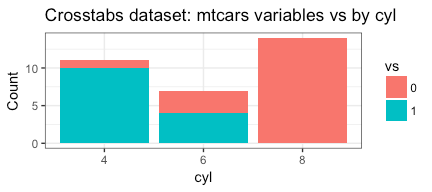```## Pairing #2 vs with gear
```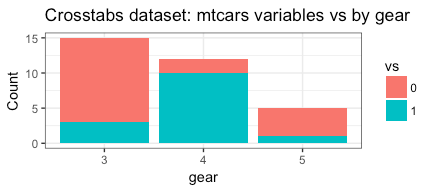```## Pairing #3 am with cyl
```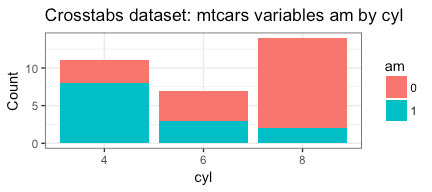```## Pairing #4 am with gear
```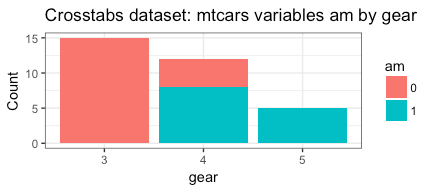```## \$dataframe
## mtcars
##
## \$xwhich
## c(8:9)
##
## \$ywhich
## c(2, 10)
##
## \$plottype
##  "stack"

PlotMeX(mtcars, 2, 8, "percent")

## Creating the variable pairings...

## Pairing #1 cyl with vs
``````## \$dataframe
## mtcars
##
## \$xwhich
##  2
##
## \$ywhich
##  8
##
## \$plottype
##  "percent"
```

For those of you who have followed his whole series of posts you’ll notice that most of what is now in the function you have seen before. It’s just reorganized. We only handle the two “clean” cases of user input all bare variables or all integers. The key differences I would note are:

```  # capture the arguments we're passed in
argList <-  as.list(match.call()[-1])
# An if test to see if both variables are bare
# if they are we'll execute immediately
if (deparse(substitute(xwhich)) %in% names(dataframe) & deparse(substitute(ywhich)) %in% names(dataframe))
# these two commands move us from column number inside the loop to bare variable name
depvarsbare <- as.name(colnames(dataframe[xwhich[[j]]]))
indvarsbare <- as.name(colnames(dataframe[ywhich[[k]]]))
```

Performance wise, let’s rerun the same `microbench` as earlier. The original mean time was ~255 milliseconds.

```# deliberately surpressing the figures!
microbenchmark(PlotMeX(mtcars, am, cyl), times = 10)

## Unit: milliseconds
##                      expr      min       lq     mean   median       uq
##  PlotMeX(mtcars, am, cyl) 9.073721 9.444969 10.52728 9.970719 10.86919
##       max neval
##  14.90618    10
```

Our new benchmark? About 9 milliseconds! Very nice! We seem to have accomplished what we set out to do. Just to be safe I also tested against the much larger (N=51020) `happy` dataset from the `productplots` package it took ~18 milliseconds.

## All done (not yet!)

This has become a very long post so I’m going to end here. Next post we’ll cleanup our code and try and address some likely issues.

I hope you’ve found this useful. I am always open to comments, corrections and suggestions.

Chuck (ibecav at gmail dot com)